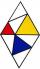# Satin

Sanusha buys a piece of satin 2.4 m wide. The diagonal length of the fabric is 4m. What is the length of the piece of satin?

Result

x =  3.2 m

#### Solution:Leave us a comment of example and its solution (i.e. if it is still somewhat unclear...):

Showing 2 comments:Zia Mcquay
woww so amazingZia Mcquay
i love this site becouse it helps alot#### To solve this verbal math problem are needed these knowledge from mathematics:

Pythagorean theorem is the base for the right triangle calculator.

## Next similar examples:

1. Tv screenThe size of a tv screen is given by the length of its diagonal. If the dimension of a tv screen is 16 inches by 14 inches, what is the size of the tv screen?
2. Diagonals in diamons/rhombusRhombus ABCD has side length AB = 4 cm and a length of one diagonal of 6.4 cm. Calculate the length of the other diagonal.
3. ParkIn the park is marked diamond shaped line connecting locations A, D, S, C, B, A. Calculate its length if |AB| = 108 m, |AC| = 172.8 m.
4. Square diagonalCalculate the length of diagonal of the square with side a = 23 cm.
5. UmbrellaCan umbrella 75 cm long fit into a box of fruit? The box has dimensions of 390 mm and 510 mm.
6. Tree trunkFrom the tree trunk, the diameter at the narrower end is 28 cm, a beam of square cross-section is to be made. Calculate the longest side of the largest possible square cross-section.
7. CablewayCableway has a length of 1800 m. The horizontal distance between the upper and lower cable car station is 1600 m. Calculate how much meters altitude is higher upper station than the base station.
8. Cone 15The radius of the base of a right circular cone is 14 inches and it's height 18 inches. What is the slant height?
9. Double ladderThe double ladder shoulders should be 3 meters long. What height will the upper top of the ladder reach if the lower ends are 1.8 meters apart?
10. Common chordTwo circles with radius 17 cm and 20 cm are intersect at two points. Its common chord is long 27 cm. What is the distance of the centers of these circles?
11. WindbreakA tree at a height of 3 meters broke in the windbreak. Its peak fell 4.5 m from the tree. How tall was the tree?
12. Double ladderThe double ladder is 8.5m long. It is built so that its lower ends are 3.5 meters apart. How high does the upper end of the ladder reach?
13. Chord circleThe circle to the (S, r = 8 cm) are different points A, B connected segment /AB/ = 12 cm. AB mark the middle of S'. Calculate |SS'|. Make the sketch.
14. Broken treeThe tree was 35 meters high. The tree broke at a height of 10 m above the ground. Top but does not fall off it refuted on the ground. How far from the base of the tree lay its peak?
15. HeightIs right that in any right triangle height is less or equal half of the hypotenuse?
16. Four ropesTV transmitter is anchored at a height of 44 meters by four ropes. Each rope is attached at a distance of 55 meters from the heel of the TV transmitter. Calculate how many meters of rope were used in the construction of the transmitter. At each attachment.
17. Three brothersThe three brothers have a total of 42 years. Jan is five years younger than Peter and Peter is 2 years younger than Michael. How many years has each of them?